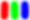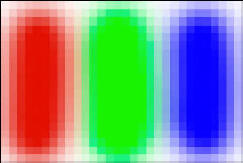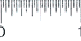'DPI' = Dots Per Inch  and  'PPI'= Pixels Per Inch and Digital Image Resolution DPI stands for Dots Per Inch. DPI represents the number of dots per linear inch to be scaled from hard copy media in preparation for a scanning job. The term DPI can also be used used to specify a printing device's native output resolution. PPI stands for Pixels Per Inch and represents the number of pixels per linear inch on a photo print when a digital image's pixels are scaled onto paper. Just like pixels, these dots can vary in their size on hardcopy media (photo prints). Mathmatically speaking, 72 dpi dots are 1/72 of an inch in diameter and 300 dpi dots are 1/300 of an inch diameter. Obviously, the dot size at 72 dpi will be much larger than the dot size at 300dpi. These two terms are relatively interchangeable. The term DPI is probably most appropriately used when you are scaling media like film or photos to be scanned to the required pixel dimensions.The term PPI is most appropriately used to scale existing digital images to a specified print size.All digital images have a IMAGE RESOLUTION (height and width dimensions in pixels). You can also specify a PRINT RESOLUTION (also called) OUTPUT RESOLUTION for a digital image by assigning and saving a PPI number within the digital image file. After assigning a PPI number your printer or imaging program does some simple math to determine the print size that would created from the existing pixel dimensions at that assigned PPI number. Assigning a print or output resolution number (PPI) SCALES the pixels in that image to specific print size based on the ratio between the assigned PPI number and existing pixel resolution of the image.A simple example would be an image with dimensions of 1200 pixels by 1800 pixels with an assigned print resolution of 300 PPI would print to 4 by 6 inches. Math formual as follows:  Height and width dimensions of the image in pixels divided by assigned PPI. 1200 pixels divided by 300 = 4 and 1800 divided by 300 = 6. See another example below= Actual image size at 20x30 pixels and .375 by .250 inch at about 80 ppi monitor resolution. The image below represents approximately what you'd get if this image was printed at 10 ppi print resolution.We will discuss scaling media for required scan resolution and scaling a digital image for required print size in more detail a bit later.

BACK - NEXT